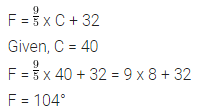# Selina Concise Mathematics Class 6 ICSE Solutions Chapter 21 Framing Algebraic Expressions

## Selina Concise Mathematics Class 6 ICSE Solutions Chapter 21 Framing Algebraic Expressions (Including Evaluation)

Selina Publishers Concise Mathematics Class 6 ICSE Solutions Chapter 21 Framing Algebraic Expressions (Including Evaluation)

### Framing Algebraic Expressions Exercise 21 – Selina Concise Mathematics Class 6 ICSE Solutions

Question 1.
Write in the form of an algebraic expression :
(i) Perimeter (P) of a rectangle is two times the sum of its length (l) and its breadth (b).
(ii) Perimeter (P) of a square is four times its side.
(iii) Area of a square is square of its side.
(iv) Surface area of a cube is six times the square of its edge.
Solution: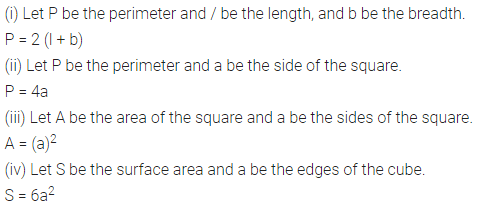Question 2.
Express each of the following as an algebraic expression :
(i) The sum of x and y minus m.
(ii) The product of x and y divided by m.
(iii) The subtraction of 5m from 3n and then adding 9p to it.
(iv) The product of 12, x, y and z minus the product of 5, m and n.
(v) Sum of p and 2r – s minus sum of a and 3n + 4x.
Solution:Question 3.
Construct a formula for the following :
Total wages (₹ W) of a man whose basic wage is (₹ B) for t hours week plus (₹ R) per hour, if he Works a total of T hours.
Solution:Question 4.
If x = 4, evaluate :
(i) 3x + 8
(ii) x2 – 2x
(iii) $$\frac { { x }^{ 2 } }{ 2 }$$
Solution: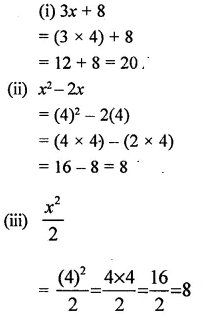Question 5.
If m – 6, evaluate :
(i) 5m – 6
(ii) 2m2 + 3m
(iii) (2m)2
Solution:Question 6.
If x = 4, evaluate :
(i) 12x + 7
(ii) 5x2 + 4x
(iii) $$\frac { { x }^{ 2 } }{ 8 }$$
Solution:Question 7.
If m = 2, evaluate :
(i) 16m – 7
(ii) 15m2 – 10m
(iii) $$\frac { 1 }{ 4 } \times { m }^{ 3 }$$
Solution:Question 8.
If x = 10, evaluate :
(i) 100x + 225
(ii) 6x2 – 25x
(iii) $$\frac { 1 }{ 50 } \times { x }^{ 3 }$$
Solution: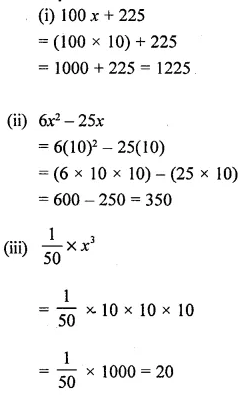Question 9.
If a = – 10, evaluate :
(i) 5a
(ii) a2
(iii) a3
Solution: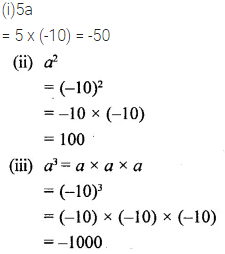Question 10.
If x = – 6, evaluate :
(i) 11x
(ii) 4x2
(iii) 2x3
Solution: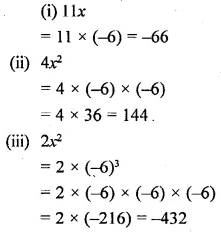Question 11.
If m = – 7, evaluate :
(i) 12m
(ii) 2m2
(iii) 2m3
Solution:Question 12.
Find the average (A) of four quantities p, q, r and s. If A = 6, p = 3, q = 5 and r = 7 ; find the value of s.
Solution:Question 13.
If a = 5 and b = 6, evaluate :
(i) 3ab
(ii) 6a2b
(iii) 2b2
Solution: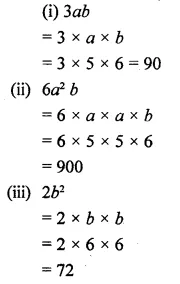Question 14.
If x = 8 and y = 2, evaluate :
(i) 9xy
(ii) 5x2y
(iii) (4y)2
Solution: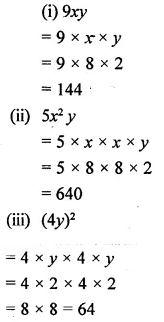Question 15.
If x = 5 and y = 4, evaluate :
(i) 8xy
(ii) 3x2y
(iii) 3y2
Solution: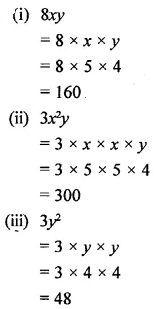Question 16.
If y = 5 and z = 2, evaluate :
(i) 100yz
(ii) 9y2z
(iii) 5y2
(iv) (5z)3
Solution: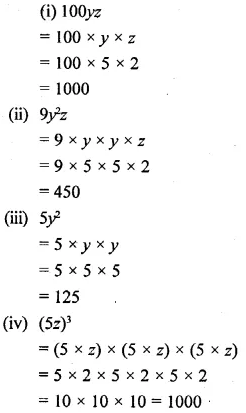Question 17.
If x = 2 and y = 10, evaluate :
(i) 30xy
(ii) 50xy2
(iii) (10x)2
(iv) 5y2
Solution: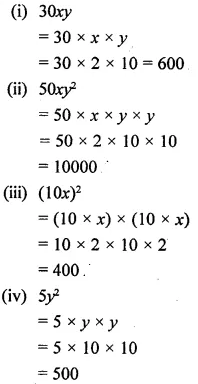Question 18.
If m = 3 and n = 7, evaluate :
(i) 12mn
(ii) 5mn2
(iii) (10m)2
(iv) 4n2
Solution: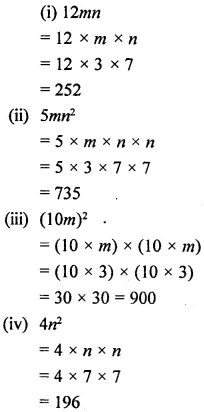Question 19.
If a = -10, evaluate :
(i) 3a – 2
(ii) a2 + 8a
(iii) $$\frac { 1 }{ 5 }$$ x a2
Solution: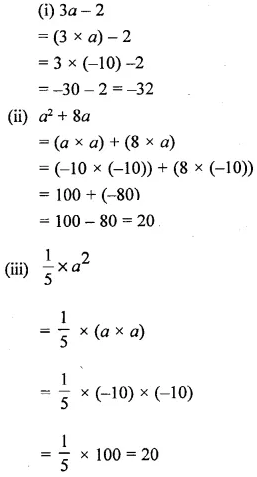Question 20.
If x = -6, evaluate :
(i) 4x – 9
(ii) 3x2 + 8x
(iii) $$\frac { { x }^{ 2 } }{ 2 }$$
Solution: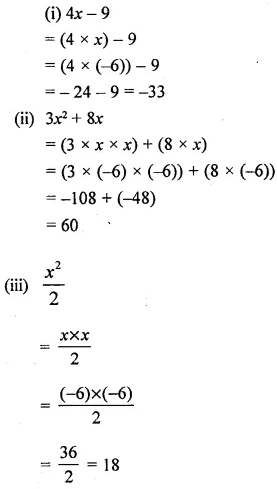Question 21.
If m = -8, evaluate :
(i) 2m + 21
(ii) m2 + 9m
(iii) $$\frac { { m }^{ 2 } }{ 4 }$$
Solution: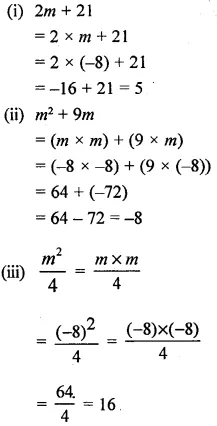Question 22.
If p = -10, evaluate :
(i) 6p + 50
(ii) 3p2 – 20p
(iii) $$\frac { { p }^{ 2 } }{ 50 }$$
Solution:Question 23.
If y = -8, evaluate :
(i) 6y + 53
(ii) y+ 12y
(iii) $$\frac { { y }^{ 3 } }{ 4 }$$
Solution:Question 24.
If x = 2 and 7 = -4, evaluate :
(i) 11xy
(ii) 5x2y
(iii) (5y)2
(iv) 8x2
Solution: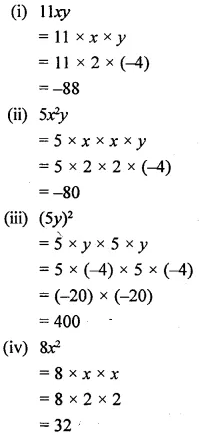Question 25.
If m = 9 and n = -2, evaluate
(i) 4mn
(ii) 2m2n
(iii) (2n)3
Solution: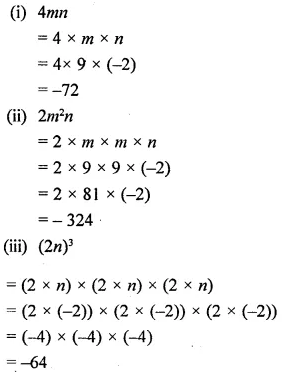Question 26.
If m = -8 and n = -2, evaluate :
(i) 12mn
(ii) 3m2n
(iii) (4n)2
Solution:Question 27.
If x = -5 and y = -8, evaluate :
(i) 4xy
(ii) 2xy2
(iii) 4x2
(iv) 3y2
Solution: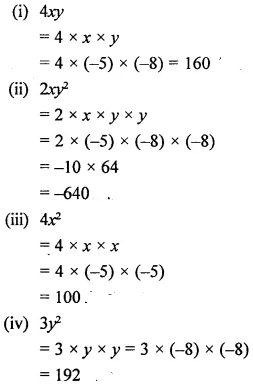Question 28.
Find T, if T = 2a – b, a = 7 and b = 3.
Solution: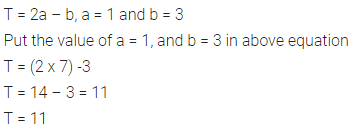Question 29.
From the formula B = 2a2 – b2, calculate the value of B when a = 3 and b = -1.
Solution:Question 30.
The wages ₹ W of a man earning ₹ x per hour for t hours are given by the formula W = xt. Find his wages for working 40 hours at a rate of ₹ 39.45 per hour.
Solution: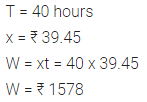Question 31.
The temperature in Fahrenheit scale is represented by F and the tempera¬ture in Celsius scale is represented by C. If F = $$\frac { 9 }{ 5 }$$ x C + 32, find F when C = 40.
Solution: